Showing first {{hits.length}} results of {{hits_total}} for {{searchQueryText}}{{hits.length}} results for {{searchQueryText}}

Attribute-value matrices are a practical way to present feature structures as a group of attributes and their corresponding values. It is possible to typeset tribute-value matrices (AVMs) with LaTeX by means of the package avm. This article explains its main features. Contents 1 Introduction 2 Basic usage 3 Unaligned columns 4 Sort labels 5 Reference guide 6 Further reading  Introduction To create an attribute-value matrix is simple, it should be typeset inside the avm environment. \documentclass{article} \usepackage[utf8]{inputenc} \usepackage[T1]{fontenc}   \usepackage{avm}   \begin{document}   \begin{avm} $cat\|subcat & \ \\ content & \[ relation & \bf bother\\ bothered & \@2 \\ soa-arg & \@3$ \] \end{avm}   \end{document}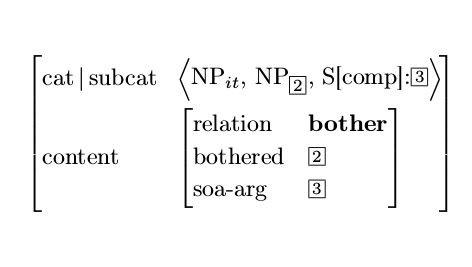The AVM package is not part of ShareLaTeX by now, but it can still be easily imported by uploading it to the root directory.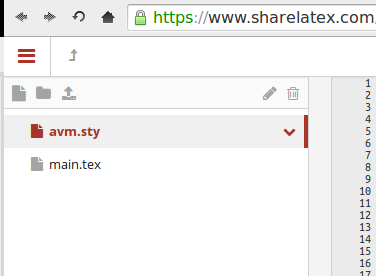Once the package is in place, it must be imported into the main file with \usepakcage{avm} This will make available the environment avm which should contain the attribute-value matrix itself. The syntax to typeset a matrix is very similar to that of an array or a tabular environment. More details and examples are presented in the subsequent sections.   Open an example of the avm package in ShareLaTeX  Basic usage To typeset an attribute-value matrix inside an avm environment there are four special commands to have in mind: \documentclass{article} \usepackage[utf8]{inputenc} \usepackage[T1]{fontenc}   \usepackage{avm} \avmfont{\sc}   \begin{document} \begin{avm} $subj & \[ pers & 3 \\ num & sg \\ gend & masc\\ pred & \rm ‘pro’$\\   pred & \rm ‘eat\q’\\   obj & $pers & 3 \\ num & pl \\ gend & fem \\ pred & \rm ‘pro’$ \] \end{avm}   \end{document}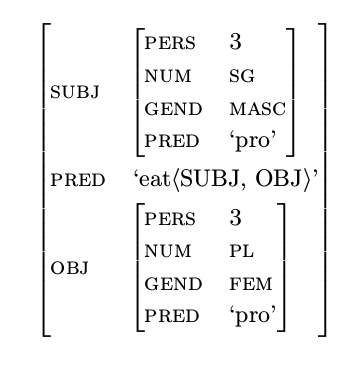We have the commands: \avmfont{\sc} Changes the font used in the matrices, in this case to small caps. Any other font family will work. The font size and font typeface can also be declared here. \begin{avm} and \end{avm} These open and close the environment where the attribute-value matrix will be created. $and$ Prints the squared brackets that delimit parts of the matrix. Other brackets can also be printed with the commands \<, \>, \{ and \} \\ Inserts a new line. & Creates a column division within the same line.   Open an example of the avm package in ShareLaTeX  Unaligned columns Sometimes, when a row is too long, it may be convenient to use unaligned columns for a better output. \begin{avm} $\avmspan{system\|local\|content\; \@2} \\ qstore & \[det & \textnormal{forall} \\ restpar & \@2 \[para & \@1 \\ restr & \[reln & \textnormal{book} \\ inst & \@1$ \] \] \] \end{avm}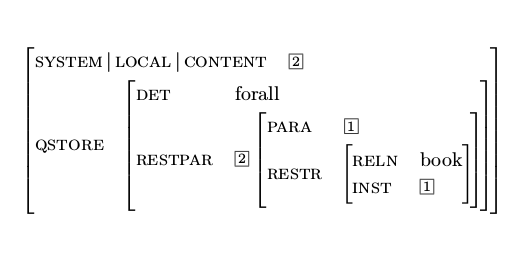The command \avmspan{} prevents column alignment for its argument whilst the command \; inserts a horizontal blank space slightly longer than normal, creating a nice separator.   Open an example of the avm package in ShareLaTeX  Sort labels It is possible to add sort labels at the corners of a AVM. There are two possible styles. The first one is printing the labels inside the matrix. \avmsortfont{\it} \avmvalfont{\it} \begin{avm} $\asort{indexed-obj} index & \[\asort{index} variable & \@1 \[\asort{variable} per & 3rd \\ num & sing \\ gend & neut$ \\ restriction & $\asort{psoa} relation & book \\ instance & \@1$ \] \] \end{avm}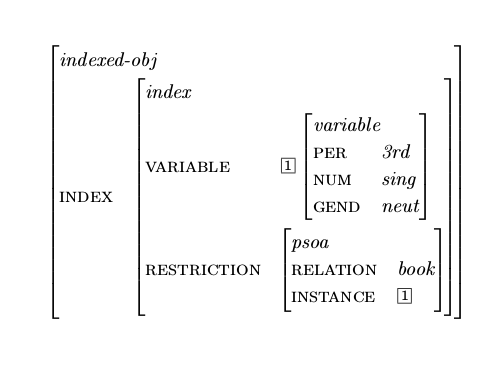There are three new commands in this example: \avmsortfont{\it} Sets the font type for the sort keys, italics in this case but other font styles and sizes can be used \avmvalfont{\it} Sets the font type for the values in the AVM, italics in this case. \asort{} Prints the sort key It's also possible to overlap the sort label onto the previous column. \avmvalfont{\it} \avmsortfont{\it} \begin{center} \begin{avm} \sort{indexed-obj}{$index & \osort{index}{\[ variable & \@1 \osort{variable}{\[ per & 3rd \\ num & sing \\ gend & neut$} \\ restriction & \osort{psoa}{$relation & book \\ instance & \@1$} \]} \]} \end{avm}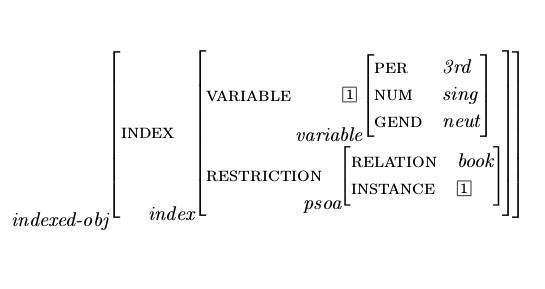There are two new commands: \sort{} Prints the label taking up space in the column where it appears. \osort{} Overlaps the label without checking collisions or available space.   Open an example of the avm package in ShareLaTeX  Reference guide Available brackets in the avm package. Command Output \{ \}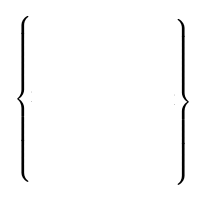\< \>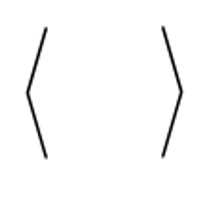Further reading For more information see The avm package documentation The avm.sty source code Font sizes, families, and styles Font typefaces Tables Brackets and Parentheses